# Statistics and Probability   Share

## What is Statistics and Probability

In simple words, Probability is the chance of happening of an event. The concept of probability is used to predict the likeliness of an event. The probability of an event lies between 0 and 1, and the higher the probability, the more likely that event will happen. For instance, the probability of getting Head in flipping a coin is ½ or 50 %.

Statistics is a branch of mathematics dealing with the collection, analysis, interpretation, and presentation of masses of numerical data.

## Overview:

Why Statistics and Probability?

In daily life, we always try to make the best decisions in which we think will succeed. Probability and Statistics give you one way to think about the decisions you make and gives you one direction to think about your decision. Using Statistics to record and analyze data, finding out probabilities for different situations or cases, and then try to make out the best rational decision possible, this method is used by all scientists, engineers, companies, and gamblers.

Prepare Online for JEE Main/NEET

Crack JEE 2021 with JEE/NEET Online Preparation Program

Today statistics and  Probability is used in all sports in making strategies, also analyzing the performance of sportsperson. For example, Average, Strike rate in Cricket and Average Goals per match in football and baseball, and all the numerical data you see in the newspaper.

Have you ever wondered,‘How Google Maps works?’

Google Maps gives you real-time traffic updates. It also tells you the shortest distance and time that you will take to reach your destination.

Google maps collect your data, like a time you take to go through a place, the distance you travel and your path between two locations, through many ways like Satellite, GPS, Location Services on your phone and Google Map Makers. At the same time, Google is collecting huge amounts of data from other people around you or going through the path you want to go. Then google analyzes all these data statistically and gives you the most probable time, distance with different routes, mode of transportation you will take between the two locations.

Sometimes Google Maps is wrong, you have to consider a fact that earth is big. With Collection of more and more data, Statistical Analysis becomes better and better, so does google maps.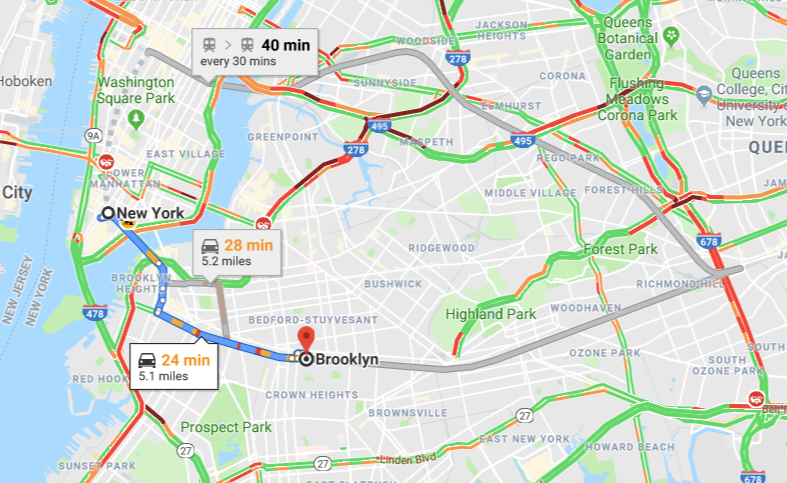Statistics and Probability for Career:

If you are preparing for entrance exams like SATs, JEE(main, advanced) or preparing for GMAT,  GRE, Probability, and Statistics cover an important portion of mathematics syllabus. Alsothere is a lot of career scope in Probability and Statistics as all companies hire data analysts to work efficiently and to improve.

## Some Basic Concepts

The basic formula of probability Theoretical Probability =(Number of Favourable Cases/Total number of possible cases)

For Example

1. Getting a probability of getting 4 or 6 in rolling a 6 faced dice is ? as the number of favorable cases is 2, i.e., {4,6} and total number of possible cases are 6, i.e, {1,2,3,4,5,6}.

2. My friend Paul wanted to bet with me that Messi will score a goal in the next match or not. He had 8 goals in 10 matches. So Paul said he surely will. I am not a big Messi fan and I did not want to biased so I used Statistics and Probability. Although he had 8 goals, he scored that in 3 matches(3,2,3) and no goals in rest 7 matches. Theoretical probability I get is 0.3. So I decided to bet against it. What would you do if you were in my situation?

Independent events:

Two or more events are said to be independent if the occurrence or non-occurrence of any of them does not affect the probability of occurrence or non-occurrence of other events. In other words, two or more events are said to be independent if occurrence or non-occurrence of any of them does not influence the occurrence or non-occurrence of other events.

For example, when a coin is tossed twice, the event of the occurrence of the head in the first throw and the event of the occurrence of the head in the second throw are independent events.Dependent events

Suppose we have 10 green balls and 10 orange balls in a box. We pull out(draw) one ball, which may be green or orange. Now there are 19 balls left in the box. What is the probability that the second ball will be orange?

It depends, If the first ball was orange, then the bag is left with 9 orange balls out of 19 so the probability of drawing an orange ball on the second draw is 9/19.  But if the first marble we draw is green, then there are still 10 orange balls in the bag and the probability of drawing an orange ball out of the bag is 10/19.

A second draw is a dependent event as we can see it depends upon the first draw.

## Conditional probability :

If A and B are two events associated with the same sample space of a random experiment, then the conditional probability of the event A under the condition that the event B has occurred. In other words, event B has already happened, now what is the chance of event A?

Conditional Probability is written as P (A | B), and it is given by

Let us understand this by taking one example, when a die is thrown, it's sample space S = {1, 2, 3, 4, 5, 6}. Let event A is an occurrence of a number greater then 4 is {5, 6}. the event of the occurrence of an even number is B = {2, 4, 6}. Then P(A|B) is the probability of occurrence of a number greater than 4, when an even number has occurred i.e. one of 2, 4, 6 has already occurred. out of these three numbers 2, 4, 6, only 6 is greater than 4. Hence, here when an even number has occurred, the total number of cases is only 3 (not 6) and favorable number of cases is only one because out of 2, 4, 6 only 6 is greater than 4.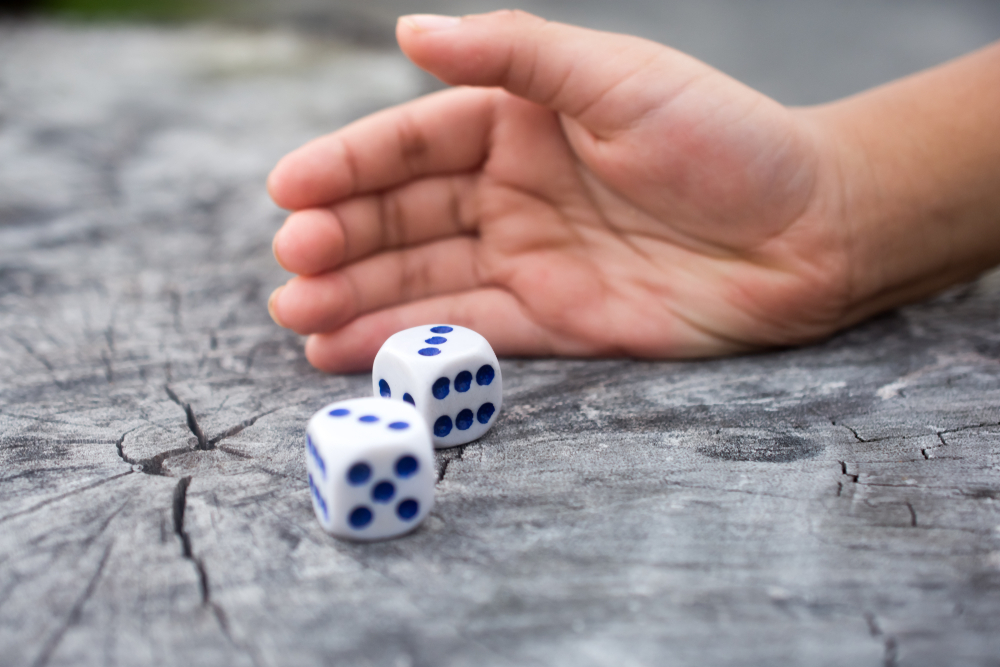## Applications of Probability

Let’s talk about the billion-dollar card game: Poker.

Poker is a card game which includes decision making based on probability, psychology and game theory. What is the probability of dealing a pair of aces in Texas Holdem Poker(dealer dealt 2 cards to all the player)?

( 4/52 )*( 3/52 ) = (12/2652) = 0.45% (approx.)

To put this in perspective, if you’re playing poker at your local casino and are dealt 25 hands per hour, you can expect to receive pocket Aces an average of once every 9 hours.

Poker professionals use all combinations of cards and their probabilities that can help in the game. Even a small change in probability can lead them to call, raise or fold. Not only poker, in all card games, including blackjack, rummy, patience, Probability, and Statistics are also used to make the game easier.

## How to study Statistics and Probability?

There are multiple ways of studying Probability and Statistics. In this section, I have written the most preferred way of understanding probability. I recommend you to study Probability before Statistics since there are applications of probability in statistical analysis.

You can start from Algebra of Events(Boolean Algebra). Then you can move to learn Probability using Venn diagrams. In that, you will learn the Basic Probability Formulas and their applications.

I would recommend you to study Permutations and Combinations or Combinatorics before studying Probability or start the next section after Venn diagrams. Permutations and Combinations or Combinatorics is a segment of mathematics concerned with counting. Do  Combinatorics based problems in probability. Practice till you have command on this topic as it is the most basic part of Probability. Also, practice applications of probability in other section of mathematics.

After that, you can move to Random Variables, Conditional Probability and Expectations, and Infinite Sequences.

For statistics, Start from definitions of mean, mode, median and then learn to mean, mode, median for grouped and ungrouped data.

## Books Recommended for Statistics and Probability:

1. NCERT Book for Class 11 (Chapter 16).

2. NCERT Book for Class 12(Chapter 13).

3. Basic Probability Theory (Robert B. Ash, Department of Mathematics, University of Illinois)

4. Head First Statistics by Griffiths.

5. Intermediate mathematical statistics Book by G. P. Beaumont

6. Introduction to the Theory of Statistics by Alexander M Mood

## Maths Chapter-wise Notes for Engineering exams

 Chapters Chapters Name Chapter 1 Sets, Relations, and Functions Chapter 2 Complex Numbers and Quadratic Equations Chapter 3 Matrices and Determinants Chapter 4 Permutations and Combinations Chapter 5 Binomial Theorem and its Simple Applications Chapter 6 Sequence and Series Chapter 7 Limit, Continuity, and Differentiability Chapter 8 Integral Calculus Chapter 9 Differential Equations Chapter 10 Coordinate Geometry Chapter 11 Three Dimensional Geometry Chapter 12 Vector Algebra Chapter 14 Trigonometry Chapter 15 Mathematical Reasoning Chapter 16 Mathematical Induction

### Topics from Statistics and Probability

• Probability of an event, addition and mulitiplication theorems of probabilty ( JEE Main, KCET, SRMJEEE, MET, COMEDK UGET, KEAM ) (120 concepts)
• Baye's theorem, probability distribution of a random variate ( JEE Main, KCET, SRMJEEE, MET, COMEDK UGET, KEAM ) (24 concepts)
• Bernoulli trials and binomial Distribution ( JEE Main, KCET, SRMJEEE, MET, COMEDK UGET, KEAM ) (24 concepts)
• Measures of dispersion, calculation of mean, median, mode of grouped and ungrouped data calculation standard deviation ( JEE Main, KCET, SRMJEEE, MET, COMEDK UGET, KEAM ) (128 concepts)
• Variance and mean deviation for grouped and ungrouped data ( JEE Main, KCET, SRMJEEE, MET, COMEDK UGET, KEAM ) (32 concepts)
• Statistics ( JEE Main, KCET, SRMJEEE, MET, COMEDK UGET, KEAM ) (40 concepts)
• Probability ( JEE Main, KCET, SRMJEEE, MET, COMEDK UGET, KEAM ) (34 concepts)

### Important Books for Statistics and Probability

•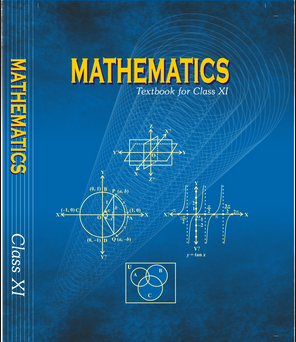•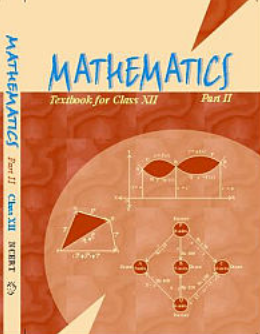•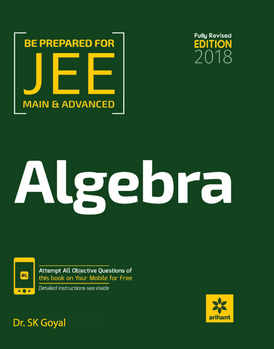Exams
Articles
Questions